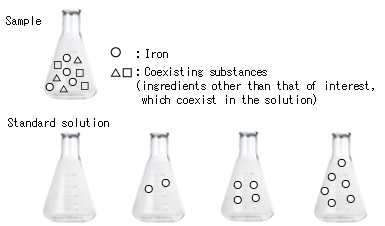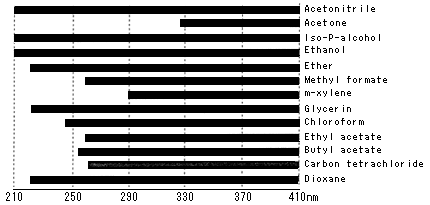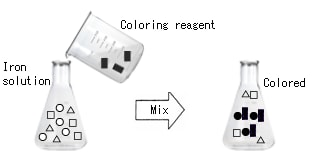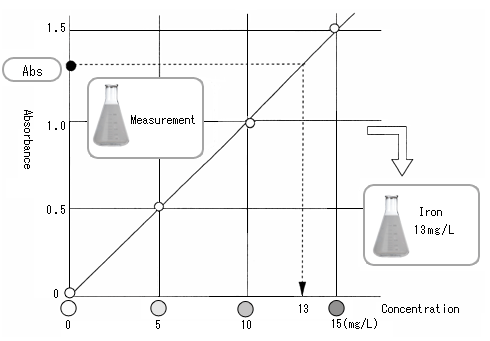# 4. Colorimetric Analysis (3)

## Measurement by color strength (quantitative analysis)

A quantitative analysis refers to an assay to measure the content of a certain substance (in a solution state).
For example, the water contains metals, small quantities of agricultural chemicals, microorganisms, and so forth.
However, the amounts contained cannot be determined visually.
Generally, in absorptiometry where a coloring reagent is added to a sample to see the degree of coloring in the reaction with the substance of interest, we use a method consisting of preparing a standard solution and a sample, adding the reagent for coloring the substance of interest, and comparing the absorbance between the standard solution and the sample using a calibration curve to determine the sample concentration.

Let's think about the procedures for measuring iron in tap water as an example.

### Sample and standard solution

To measure the concentration of a substance, a sample of unknown concentration to be measured ("sample") is compared with a sample of known concentration ("standard solution") to determine the concentration. To analyze iron in tap water, tap water is used as a sample, and iron of known concentration as a standard solution. Standard solutions of different iron concentrations are prepared as standard solutions.

Accurate results cannot be obtained unless the sample and the standard solution are prepared and measured under the same conditions. For example, when the sample is tap water, an aqueous solution is used as a standard solution instead of an alcoholic solution; when an acid is added to the sample, the acid should be added also to the standard solution.A liquid that dissolve a substance, such as water and alcohol, is called "solvent," and a substance that is dissolved into a liquid, such as iron, is called "solute." Accurate results cannot be obtained when absorption occurs at the same wavelength for the solute and the solvent.

Generally, water is often used since there is no absorption in the visible-ultraviolet range. An organic solvent is also used commonly; however, take care about its use due to absorption in the invisible ultraviolet range.

Use of an organic solvent in the ultraviolet range Water is used as a control, with the absorbance of 1 or less.### Magic drug (coloring reagent)

Can you see the color of iron in tap water? Colorimetric analysis is a method to analyze a color. The assay is impossible if a color cannot be seen (in the case of visible ranges). Then, what should be done.....? Color iron with a magic drug.

The magic drug that colors the substance of interest in a solution is called "coloring reagent" or "color reagent."

The higher the iron content in tap water, the darker becomes the color (high absorbance). The lower the iron content in tap water, the lighter becomes the color (low absorbance). Since various color reagents are available for each component, they can be used according to the analytical components.### Calibration curve

Measure the absorbance of a standard solution to prepare a calibration curve. The horizontal axis indicates concentration, and the vertical axis absorbance. By measuring standard solutions in the order of iron concentrations (from a low concentration), and plotting the concentrations and absorbance, we obtain the graph shown in the figure below.

This is the "calibration curve."

When the standard solution is measured and the calibration curve is prepared, measure a sample. Measurement provides absorbance, although the concentration is unknown. See the calibration curve. The absorbance of the vertical axis provides the concentration.

In a quantitative analysis, the concentration of a sample is determined in this manner.